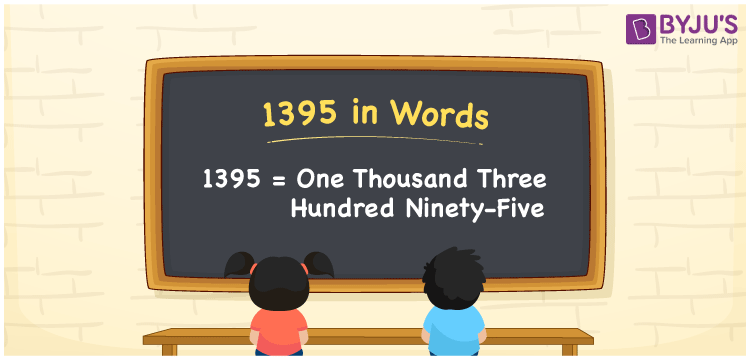# 1395 in Words

We can write 1395 in words as One Thousand Three Hundred Ninety-five. Suppose you have Rs. 1395 in your bank account, you can say that “I have One Thousand Three Hundred Ninety-five rupees in my bank account”. It is possible to convert 1395 into words with the help of a place value chart. Also, we know that 1395 is a cardinal number since it represents a specific quantity.

 1395 in words One Thousand Three Hundred Ninety-five One Thousand Three Hundred Ninety-five in Numbers 1395

## 1395 in English Words## How to Write 1395 in Words?

We can convert 1395 to words using a place value chart. This can be done as follows. The number 1395 has 4 digits, so let’s make a chart that shows the place value up to 4 digits.

 Thousands Hundreds Tens Ones 1 3 9 5

Thus, we can write the expanded form as:

1 × Thousand + 3 × Hundred + 9 × Ten + 5 × One

= 1 × 1000 + 3 × 100 + 9 × 10 + 5 × 1

= 1395

= One Thousand Three Hundred Ninety-five

1395 is the natural number that is succeeded by 1394 and preceded by 1396.

1395 in words – One Thousand Three Hundred Ninety-five

Is 1395 an odd number? – Yes.

Is 1395 an even number? – No

Is 1395 a perfect square number? – No

Is 1395 a perfect cube number? – No

Is 1395 a prime number? – No

Is 1395 a composite number? – Yes

## Frequently Asked Questions on 1395 in Words

Q1

### How to write 1395 in words?

1395 in English is written as “One Thousand Three Hundred Ninety-five”.
Q2

### Is 1395 a prime number?

No, the number 1395 is not a prime number. One Thousand Three Hundred Ninety-five is a composite number. Because 1395 has more divisors than 1 and itself.
Q3

### Write One Thousand Three Hundred Ninety-five in numbers.

One Thousand Three Hundred Ninety-five in numbers is 1395.